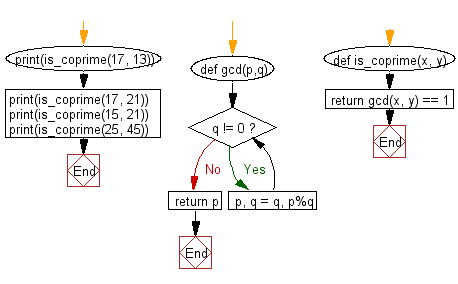﻿ Python: Check if two given numbers are Co Prime or not - w3resource# Python: Check if two given numbers are Co Prime or not

## Python Basic - 1: Exercise-119 with Solution

Two numbers are coprime if their highest common factor (or greatest common divisor if you must) is 1.
Write a Python program to check if two given numbers are Co Prime or not. Return True if two numbers are Co Prime otherwise return false.

Sample Solution:

Python Code:

``````def gcd(p,q):
# Create the gcd of two positive integers.
while q != 0:
p, q = q, p%q
return p
def is_coprime(x, y):
return gcd(x, y) == 1
print(is_coprime(17, 13))
print(is_coprime(17, 21))
print(is_coprime(15, 21))
print(is_coprime(25, 45))
``````

Sample Output:

```True
True
False
False
```

Flowchart:Python Code Editor:

Have another way to solve this solution? Contribute your code (and comments) through Disqus.

What is the difficulty level of this exercise?

Test your Programming skills with w3resource's quiz.

﻿

## Python: Tips of the Day

What is the difference between Python's list methods append and extend?

append: Appends object at the end.

```x = [1, 2, 3]
x.append([4, 5])
print (x)
```

Output:

```[1, 2, 3, [4, 5]]
```

extend: Extends list by appending elements from the iterable.

```x = [1, 2, 3]
x.extend([4, 5])
print (x)
```

Output:

```[1, 2, 3, 4, 5]
```

Ref: https://bit.ly/2AZ6ZFq